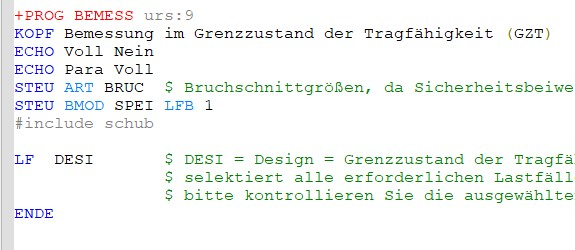# Flächenbemessung GZT

For calculating the reinforcement of a plate, one should define the loadcase LF for the calculation - in this case DESI for the GZT; some rows above I have defined only one combination DESI (2100), which will be taken for plate calculation.

1. What if I have three DESI combinations (2100,3100,4100)? Which one will be considered for Plate calc.?

2. How can I chose only one combination for the plate calculation?1. all cases with `desi` action. you can check list of cases used to design in report browser.
2. you can do it in many ways:
• use diffrent action for each combinations in maxima, e.g. d_1, d_2, d_3 etc (but create it before in sofiload), then in bemess e.g. `lc d_1`
• write list of cases instead of action name, e.g. `lc (2101 2199 1)` or `lc 2101,2102,2151`